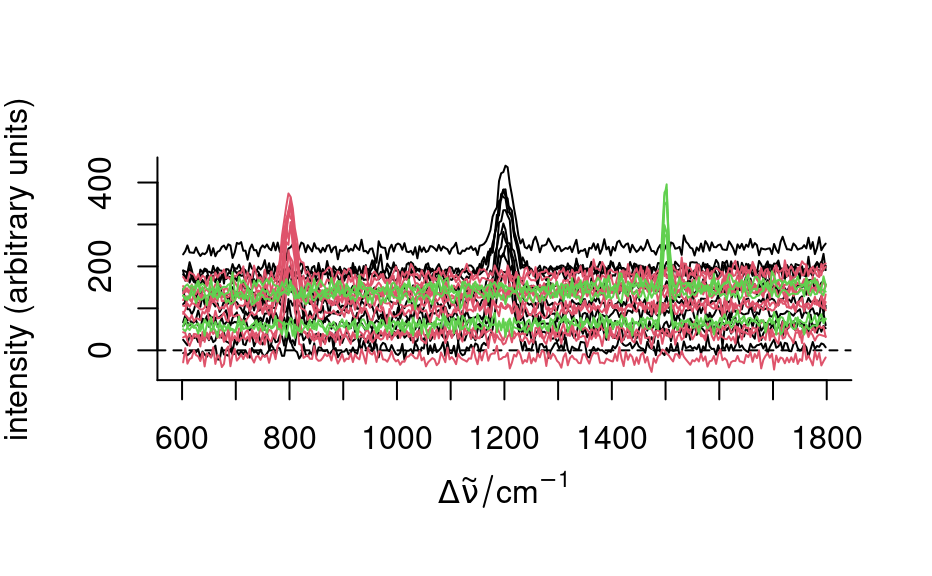Interface functions to use mvtnorm::rmvnorm() for hyperSpec objects.

rmmvnorm(n, mean, sigma)

# S4 method for numeric,hyperSpec,matrix
rmmvnorm(n, mean, sigma)

# S4 method for numeric,hyperSpec,array
rmmvnorm(n, mean, sigma)

# S4 method for numeric,matrix,matrix
rmmvnorm(n, mean, sigma)

# S4 method for numeric,matrix,array
rmmvnorm(n, mean, sigma)

Arguments

n

vector giving the number of cases to generate for each group.

mean

matrix with mean cases in rows.

sigma

common covariance matrix or array (ncol(mean) x ncol(mean) x nrow(mean)) with individual covariance matrices for the groups.

Details

The mvtnorm() method for hyperSpec objects supports producing multivariate normal data for groups with different mean but common covariance matrix, see the examples.

• mvtnorm::rmvnorm() (with 1 'm') allows a single covariance matrix.

• hyperSpec::rmmvnorm() (with 2 'm') allows multiple covariance matrices for multiple groups. It has methods not only for hyperSpec objects but also such a multi-group extension for numeric objects.

mvtnorm::rmvnorm()

cov() and cov_pooled() about calculating covariance of hyperSpec objects.

Examples

## multiple groups, common covariance matrix

if (require("mvtnorm")) {
pcov <- cov_pooled(faux_cell, faux_cell$region) rnd <- rmmvnorm(rep(10, 3), mean = pcov$mean, sigma = pcov$COV) plot(rnd, col = rnd$.group)
}